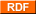# Cambalache 3,14 - La vidriera irrespetuosa

Que el mundo fue y será una porquería, ya lo sé.

### 53 (advanced post)

Viene de esta otra anotación sobre el número 53
is the smallest prime which is not the difference between powers of 2 and 3.

is the only two-digit number that is reversed in hexadecimal (base 16): 3516

xy + x + y never yelds 53.

the reciprocal of 53 (1/53) has 13 digits that repeat: 0.01886792452830188679245283....

is the sum of five consecutive primes: 5 + 7 + 11 + 13 + 17

the sum of the first 53 integers is 1431, which is divisible by 53, a property shared by many other numbers.

the sum of the first 53 primes is 5830, which is divisible by 53, a property shared by few other numbers.

the sum of all consecutive prime numbers from 7 to 53 is the product of 7 x 53:
7 x 53 = 7+11+13+17+19+23+29+31+37+41+43+47+53 = 371

53 = 22 + 72 = 12 + 42 + 62 (sum of squares)
532 = 282 + 452
535 = 418195493 = (4+18+1+9+5+4+9+3)5
537 = 1174711139837 = (1+1+7+4+7+1+1+1+3+9+8+3+7)7

253 = 9007199254740992 is the smallest power of 2 containing two successive zeroes.

the chance that no pair of 53 people in a room have the same birthday is approximately 1/53.

is the code for international direct dial phone calls to Cuba.

is the number of countries in Africa.
via What's Special About This Number?

2013-09-23 15:06 | Categoría: | Enlace permanente | Etiquetas: | Y dicen por ahí

URL de trackback de esta historia http://zifra.blogalia.com//trackbacks/73811

### Busca en Cambalacheweb metrics

### Archivos:

Lu Ma Mi Ju Vi Sa Do < Abril 2021 1 2 3 4 5 6 7 8 9 10 11 12 13 14 15 16 17 18 19 20 21 22 23 24 25 26 27 28 29 30

### FotoFlickr

2003-2006 Zifra Powered by Blogalia